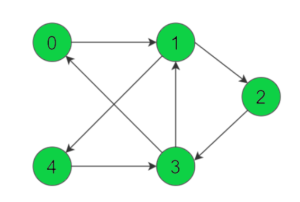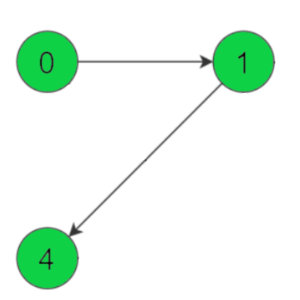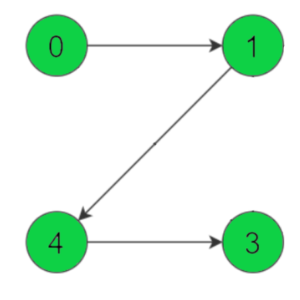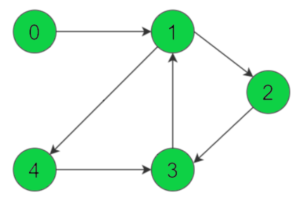In this article, will look at an interesting algorithm related to Graph Theory: Hierholzer’s Algorithm. We will discuss a problem and solve it using this Algorithm with examples. We will also discuss the approach and analyze the complexities for the solution.

Hierholzer’s Algorithm has its use mainly in finding an Euler Path and Eulerian Circuit in a given Directed or Un-directed Graph. Euler Path (or Euler Trail) is a path of edges that visits all the edges in a graph exactly once. Hence, an Eulerian Circuit (or Cycle) is a Euler Path which starts and ends on the same vertex.

Let us understand this with an example, Consider this Graph :In the above Directed Graph, assuming we start from the Node 0 , the Euler Path is : 0 -> 1 -> 4 -> 3 -> 1 -> 2 and the Eulerian Circuit is as follows : 0 -> 1 -> 4 -> 3 -> 1 -> 2 -> 3 -> 0. We can see that the Eulerian Circuit starts and ends on the same vertex 0.

Note: We see some nodes being repeated in the Euler Path. It is so because the above graph is directed so we have to find a path along the edges. If the above graph was Un-directed the Path would be : 0 -> 1 -> 2 -> 3 -> 4.

### Necessary Conditions for Eulerian Circuit

Now let us look at some conditions which must hold for an Eulerian Graph to exist in a Directed Graph.

• Every vertex must have an equal In-degree and Out-degree. In-degree is the number of edges incident on a vertex. Out-degree is the number of outgoing edges from a vertex.
• There can be at most one such vertex whose Out-degree – In-degree = 1 and one such vertex whose In-Degree – Out-degree = 1.  Hence, if there are more than one such vertex, it means the Eulerian Circuit does not exist for the graph.
• All of the vertices having non-zero degree should belong to a Single Strongly Connected Component.
• Hence, The vertices which follow the second condition can act as the starting and the ending vertices of the Euler Path.

If the In and Out degrees of all vertices are equal to each other. Then any vertex can be our starting node. Generally we choose a vertex with smallest Out-degree or an odd degree vertex..

The In-Degree and Out-Degree of the vertices of the above graph is :

Node 0 -> In-Degree: 1 , Out-Degree: 1. For, Node 1 -> In-Degree: 2 , Out-Degree: 2 . Node 2 -> In-Degree: 1 , Out-Degree: 1 . For, Node 3 -> In-Degree: 2 , Out-Degree: 2. Node 4 -> In-Degree: 1 , Out-Degree: 1.

## Hierholzer’s Algorithm

Now let us look at how Hierholzer’s Algorithm is useful in finding Eulerian Circuit for the above graph.

• For the above graph, we choose Vertex 0 as starting node, follow a trail of edges from that vertex until returning back to it. It is not possible to get stuck at any vertex, because In-degree and Out-degree of every vertex is same.
• If we come again to the start vertex while all the vertices are not visited yet, we backtrack to the nearest node which has a edge to a unvisited node. We will repeat this process and follow the trail along the directed edges until we get to the starting node and then we unwind the stack and we print the nodes.
• For each node we visit we will decrement the count of its Outgoing Edges or Out-degree by 1, to ensure that we do not visit the same vertex again unless, there exists a node which has to be visited from that vertex only.

### Step-by-Step Example

Let us look at a step by step example how we use this Algorithm for the above example graph.

We start from Node 0 which is our starting node to Node 1. We will decrement the count of the source node’s outgoing edge or Out-degree after every node we visit. So Current Outdegree of Node 0 is 0. Hence, The Eulerian Path looks like :After this, we do a normal DFS Traversal for every node so we visit the node 4 and decrement Outdegree of Node 1 . So Outdegree of Node 1 is 1. The Path now is :Now, 4 has an outgoing edge to Node 3, we visit it and update its Out-degree which is now 0. Thus now the Euler path is :So, now 3 has an outgoing edge to Node 1 again, we visit it and decrement its Outdegree to 1. We do not visit node 0 because it has no pending nodes to be visited. Along with this, we have to maintain the constraint that discussed in Step 3 of Algorithm above. So the path now is :Now, Node 1 has an edge to yet unvisited node 2, we traverse to it and update it’s Outgoing edge count = 1-1 =0.  The updated Path is:Finally, Node 2 has an edge to node 3, we visit it and update its Outdegree to 0. Thereby completing the Euler Path traversing all the nodes. To complete the cycle or Eulerian Circuit we visit Node 0 from Node 3 which results the original graph.Thus, the Eulerian Circuit is : 0 -> 1 -> 4 -> 3 -> 1 -> 2 -> 3 -> 0 .

Note: There was no Back-tracking done in this example, as we visited every node once except the condition when node 2 was to be visited.

### Implementation in Java

For the implementation we use a 2D – List in Java (Vector in C++), to store the nodes along with their outgoing edges. We will use a Map (Hash-Map) to store count of outgoing edges for each vertex. The Key being the vertex and the Value will be the Out-degree of the same vertex. We use a Stack to maintain which nodes are processed at any instant. As soon as we get the Out-degree for any node equal to 0 we add it to our result array or list.

Let us look at the implementation code in JAVA:

```import java.util.*;
public class Hierholzer_Euler

public static void main(String args[])

List< List<Integer> > adj = new ArrayList<>();

// Build the Graph

System.out.println("The Eulerian Circuit for the Graph is : ");

static void printEulerianCircuit(List< List<Integer> > adj)

// the directed graph
// edge represents the number of edges emerging from a vertex

Map<Integer,Integer> edges=new HashMap<Integer,Integer>();

//find the count of edges to keep track of unused edges

// Maintain a stack to keep vertices
Stack<Integer> curr_path = new Stack<Integer>();

// vector to store final circuit
List<Integer> circuit = new ArrayList<Integer>();

// We start from vertex 0
curr_path.push(0);

// Current vertex
int curr_v = 0;

while (!curr_path.empty())

// If there's remaining edge
if (edges.get(curr_v)>0)

// Push the vertex visited.

// and remove that edge or decrement the edge count.
edges.put(curr_v, edges.get(curr_v) - 1);

// Move to next vertex
curr_v = curr_path.peek();

// back-track to find remaining circuit
else

curr_v = curr_path.pop();

// After getting the circuit, now print it in reverse
for (int i=circuit.size()-1; i>=0; i--)

System.out.print(circuit.get(i));

if(i!=0)
System.out.print(" -> ");

```

Output:

```The Eulerian Circuit for the Graph is :
0 -> 1 -> 4 -> 3 -> 1 -> 2 -> 3 -> 0```

Now, let us have a quick look at the complexities of this Algorithm.

Time Complexity: We do a modified DFS traversal, where we traverse at most all the edges in the graph to complete the Eulerian Circuit so the time complexity is O(E), for E edges in the Graph. Unlike Fleury’s Algorithm which takes O(E*E) or O(E2) time, Hierholzer’s Algorithm is more efficient.

Space Complexity: For extra Space, we use a Map and a Stack to keep track of the edges of each node and the nodes processed respectively. So we at the most store all the vertices of the Graph, so the overall complexity is O(V), where V is the number of vertices.

That’s it for the article you can try out this Algorithm with different examples and execute the code for better understanding.

Let us know your suggestions or doubts (if any) in the comments section below.

The post Hierholzer’s Algorithm with Implementation in Java appeared first on The Crazy Programmer.